# AN156 - Using a Microcontroller to Adjust the MP2659’s Charging CurrentGet valuable resources straight to your inbox - sent out once per month

## ABSTRACT

To fine-tune their performance, many battery-powered devices must adjust the charging current in real time under different operating modes. For example, many battery manufacturers require specific charging current levels for different temperatures to guarantee battery safety. So a method for charging current adjustment in real time is beneficial.

The MP2659 can regulate its charging current with a resistor (RISET) connected between the ISET pin and AGND. This application note details how to adjust the MP2659’s charging current in real time with a microcontroller (MCU), based on the charging current regulation. This application note describes the MP2659, and addresses the relationship between RISET and the charging current. Next, this note recommends a design guide for charging current real-time adjustment, and lastly provides a design example.

## 1. INTRODUCTION

The MP2659 is a highly integrated switching charger designed for applications with 3-cell to 6-cell series Li-ion or Li-polymer battery packs. It supports several battery chemistries with different battery regulation voltages. The charging current can be regulated by connecting a corresponding resistor (RISET) between the ISET pin and AGND. The relationship between RISET and the charging current can be calculated with Equation (1):

$$I_{CHG} = \frac{96(kΩ)}{R_{ISET}(kΩ)}$$

Figure 1 shows the standard schematic for the MP2659.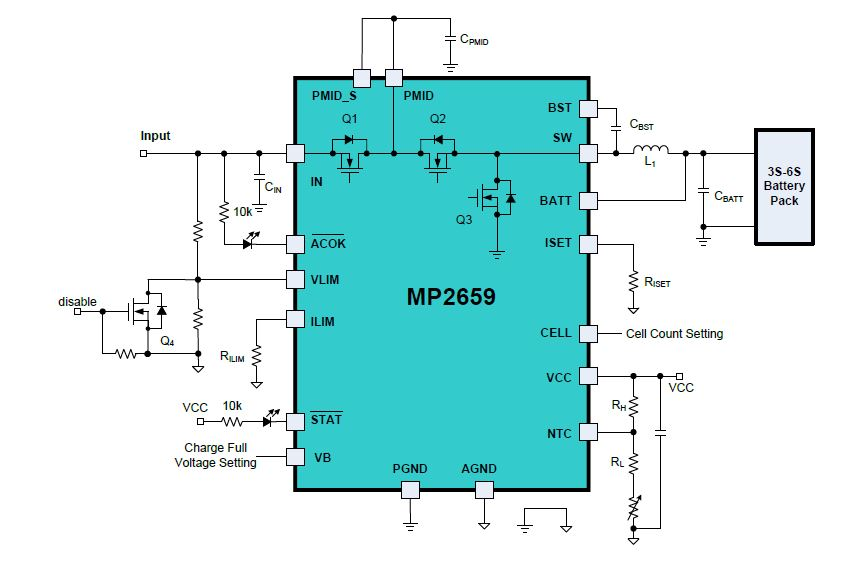Figure 1: MP2659 Application Schematic

Although the charging current can be adjusted with RISET, this method is unreliable for real-time regulation. By using the PWM of an MCU, the charging current can be adjusted in real time with greater reliability.

## 2. METHOD DETAILS

The MP2659 regulates the charging current with different resistor values read by the ISET pin. The ISET pin maintains a constant voltage of 1.2V, meaning the charging current can be adjusted by changing the equivalent resistor between the ISET pin and AGND. This change can be initiated by modifying the MCU’s PWM duty cycle.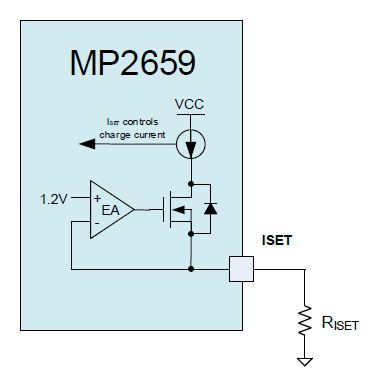Figure 2: Equivalent Circuit of the ISET Pin

### 2.1 Method Overview

Figure 3 shows how to build a design when the equivalent resistor is connected to the MP2659’s ISETpin.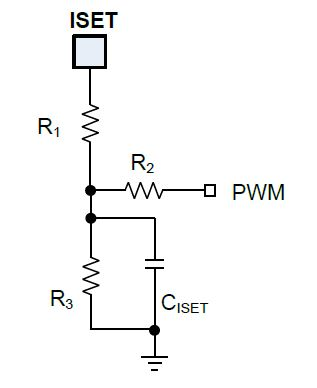Figure 3: Equivalent Resistor Design Circuit

The PWM signal has a controllable duty cycle. This signal is generated by an MCU. The RC filter (consisting of R2, R3, and CISET) filters the PWM signal to the DC signal. The equivalent resistance (REQ)between the ISET pin and AGND can be calculated with Equation (2):

$$R_{EQ}=\frac {1.2R_1G_{123}}{1.2G_{123} - (\frac{DUTY \times V_{M\_PWM}}{R_2}+\frac{1.2}{R_1})}$$

Where DUTY is the PWM duty cycle, and VM_PWM is the PWM amplitude (the same as the MCU supply voltage, which is about 3.3V). G123 can be estimated with Equation (3):

$$G_{123} = {1 \over R_1} + {1 \over R_2} + {1 \over R_3}$$

For Equations 1, 2, and 3, REQ must exceed 0Ω. When REQ falls below 0Ω, ICHG = 0A. Table 1 lists the charging current based on the PWM input.

Table 1: Charging Current vs. PWM Input

 PWM Input PWM Duty Charging Current GND 0% 96(kΩ)/(R1+R2//R3) Logic high 100% 0A PWM duty cycle 0% to MAX_DUTY (1) Linearly adjust charging current Float N/A 96(kΩ)/(R1+R2)

Note:

1) MAX_DUTY is the maximum PWM duty when the charging current falls to 0A. It is recommended to be about 80%.

### 2.2 Parameters-Related Design Guide

Based on the analysis above, the parameters (R1, R2, R3, CISET) can be designed with the following guidelines:

• The equivalent resistor that corresponds to the max charging current is RMAX_ICHG, which can be calculated with Equation (4):
• $$R_{MAX\_ICHG} = R_1 + R_2 // R_3$$
• Choose an appropriate R1, estimated with Equation (5):
• $$R_1 = 0.5R_{MAX\_ICHG}$$
• Solve the equations, then calculate R2 and R3 with Equation (6):
• $$\begin{cases} R_2//R_3=R_1 \\[2ex] \frac{MAX\_DUTY \times V_{M\_PWM} - 1.2}{R_2} = \frac{1.2}{R_3} \end{cases}$$
• Choose an appropriate CISET to filter the PWM signal to DC signal, estimated with Equation (7):
• $$f_{FILTER} = \frac {1}{2\pi(R_2//R_3)C_{ISET}} << f_{PWM}$$

Where fFILTER is the cut-off frequency of the RC filter, it is recommended to be about 10Hz; fPWM is the PWM frequency, it is recommended to be above 1kHz.

## 3. DESIGN EXAMPLE

This section demonstrates how to successfully design the parameters to adjust the charging current in real time when MAX_ICHG = 2.7A, MAX_DUTY = 80%, VM_PWM = 3.3V, and fPWM = 2kHz.

### 3.1 Design Process

1. Calculate RMAX_ICHG using Equation (8):
2. $$R_{MAX\_ICHG} = \frac {96(kΩ)}{MAX\_ICHG} = 35kΩ$$
3. Choose R1 = 20kΩ.
4. Use Equation (6) to calculate R2 = 33kΩ, and R3 = 27kΩ.
5. Set CISET = 1μF to filter the PWM signal to the DC signal.

### 3.2 Design Results

Build a demo board with the parameters determined above to verify the effectiveness of the method proposed in this application note. Figure 4 shows the demo board schematic.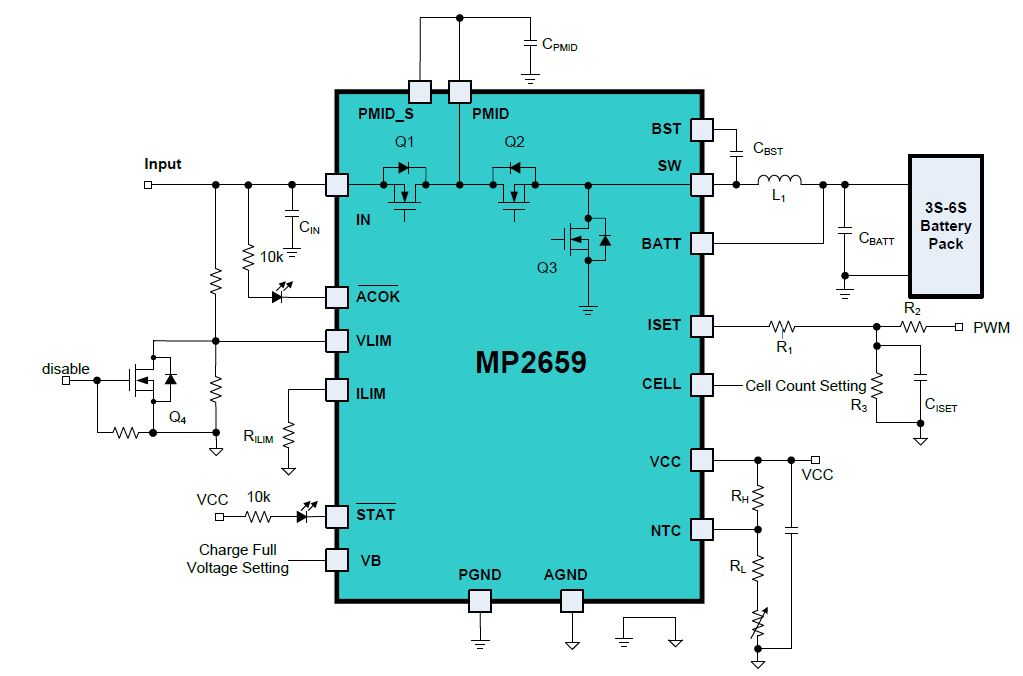Figure 4: MP2659 Charging Current Adjustment Application Demo Board Schematic

According to the results obtained after testing the demo board (under the test conditions: VIN = 16V, VBATT = 12V, VM_PWM = 3.3V, and fPWM = 2kHz), there is a linear relationship between the charging current and the PWM duty cycle, and the duty cycle offers a wide range of 0% to 82%. (see Figure 5).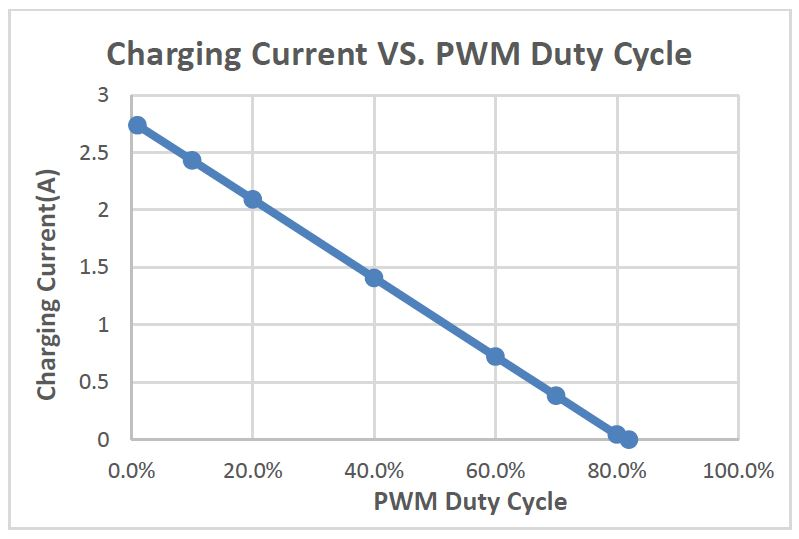Figure 5: Charging Current vs. PWM Duty Cycle

The MP2659 does not experience overshoot or undershoot when the charging current changes (see Figure 6). In addition, the device demonstrates normal start-up and shutdown (see Figure 7).

All the test results offer evidence supporting the effectiveness of the design method introduced in this application note.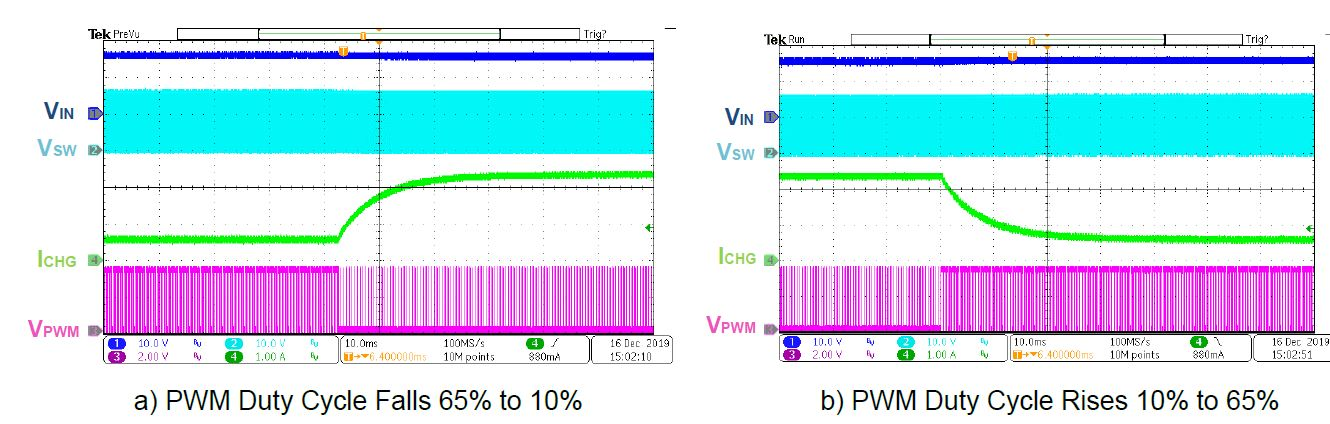Figure 6: Charging Current Transition between 0.5A and 2.4A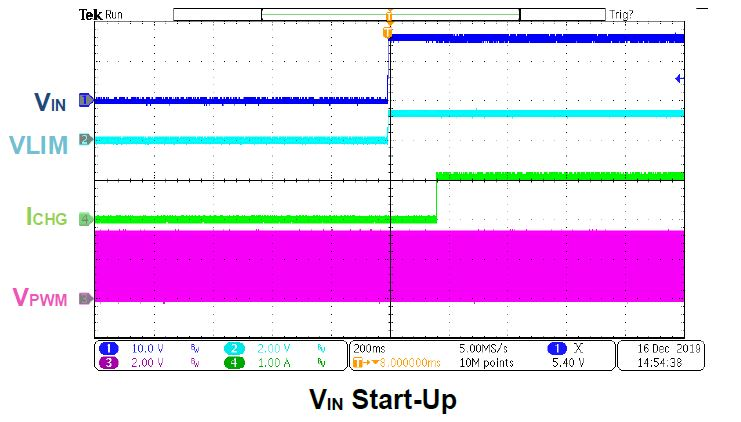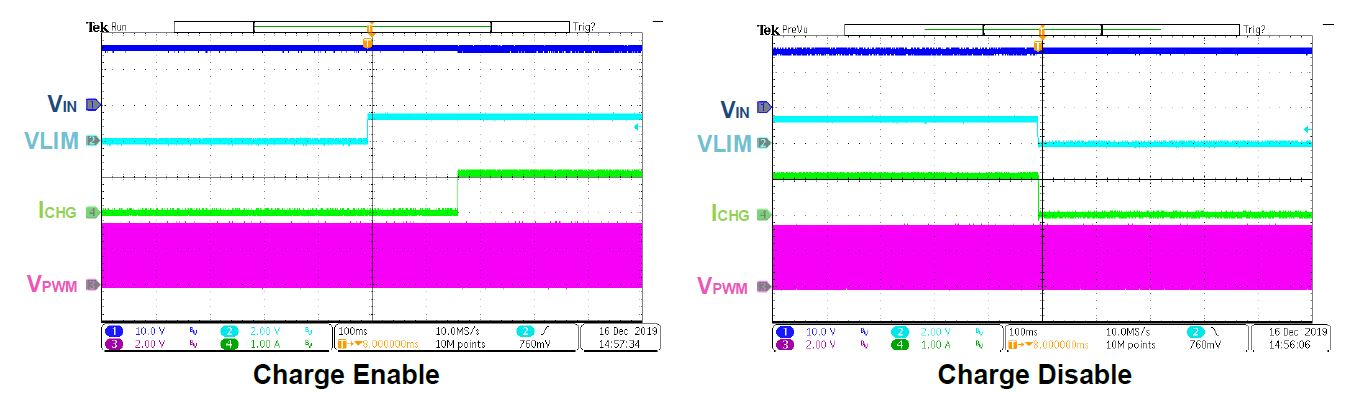Figure 7: Waveforms of MP2659 On/Off Application (PWM Duty = 50%)

## 4. CONCLUSION

Charging performance can be optimized with an adjustable charging current. The application note proposed a method to adjust the MP2659’s charging current in real time with an MCU. A design example was provided, as well as test results to prove the validity of this current-charging method.